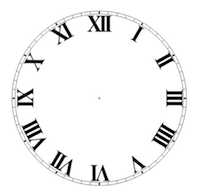December 2015 Brain Teaser SolutionNotice that 5 has an exclamation point on it.  This means factorial so that 5! = 5 x 4 x 3 x 2 x 1 = 120.

November 2015 Brain Teaser Solution

Which is greater, 2^1100 or 3^700?
Bonus: Approximately how many times greater is it (to the nearest 10)?
Both of these are too large to do on a calculator and will force the calculator into overflow.  So we have to come up  with another way to compare them rather than getting both actual values.
We can write them as:
(2^11)^100   and (3^7)^100 and just compare the
2^11   vs 3^7
2048 vs 2187
so 3^700 is the winner!!
Here is a link to the answer to the bonus
Thanks for solving

October 2015 Brain Teaser Solution

Q: How many squares are on an 8 x 8 checkerboard?  10 x 10? How many rectangles?

8 x 8 There are:
64 1 x 1
49 2 x 2
36 3 x 3
25 4 x 4
16 5 x 5
9  6 x 6
4 7 x 7
1 8 x 8 for a total of 204 squares

For 10 x 10
100 1 x 1
81 2 x 2
64 3 x 3
49 4 x 4
36 5 x 5
25 6 x 6
16 7 x 7
9 8 x 8
4 9 x 9
1 10 x 10

for a total of 385 squares

The rectangle counting is significantly more challenging.
Click here for a beautiful explanation from Nigel Coldwell

August 2015 Brain Teaser Solution

Q: You have 8 batteries but only 4 of them work and 2 are needed to power a flashlight.  What is the fewest number of pairs you need to test to guarantee you can get the flashlight on?
A: 7. If you break the batteries into 3 groups: Two groups of 3 and one group of 2. By doing this you guarantee that one of the groups has 2 working batteries. Both of the groups of 3 have 3 possible combinations of 2 batteries and the group of 2 only has 1 combination. So, 3 + 3 + 1 = 7 tries at most to find two working batteries.

June 2015 Brain Teaser Solution

Q: Can you take the clock face below, and cut it into four pieces such that the numerals on each part add up to the same number?A: The key is to turn the number XI upside down to a IX or:
turn the IX upside down to a XI
The numbers 1-12 add up to 78 which is not divisible by 4
Replace 11 with a 9 and the numbers now add up to 76 which is divisible by 4
and/or replace the 9 with an 11 and now the numbers add up to 80 which is also divisible by 4